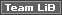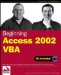# IIfWe mentioned IIf earlier in the book (Chapter 4), and warned you of its dangers (all of the arguments are run), but there are some times when it can be really useful. For example, suppose we want to print our report out double sided, and would like the page numbers to appear at the outer edge on both odd and even numbered pages. This means that for some pages it must be on the left, and for the others it must be on the right. Note that as an alternative, we could write our own function to do this: we probably would if we wanted more flexibility than the IIf function offers.

### Try It Out-The IIf Function

1. Switch the form back into design mode.

2. Move the page number field to the very left of the page, and set the text to be left aligned using the Align Left button on the toolbar.

3. Modify the Control Source property of the page number field, so it now looks like this:

` =IIf([Page] Mod 2 = 0, "Page " & [Page] & " of " & [Pages], "") `
4. Add another textbox, this time at the right of the page and set the text to be right aligned using the Align Right button on the toolbar. You can delete the label again.

5. Add the following code to this textbox's Control Source property:

` =IIf([Page] Mod 2 = 1, "Page " & [Page]  & " of " & [Pages], "") `
6. Now switch to preview mode to see what effect the changes have had. Step forward a few pages to see what happens for odd and even pages.

Notice that for the first page, and all odd numbered pages, the page numbers are on the right of the page. For all even numbers they are on the left.

How It Works

Let's look again at the arguments for the IIf function:

` IIf (Expression, TruePart, FalsePart) `

The arguments are:

• Expression , which is the expression to test

• TruePart , which is the value to return if Expression is True

• FalsePart , which is the value to return if Expression is False

So, for the page numbers on the left we have this:

` =IIf([Page] Mod 2 = 0, "Page " & [Page] & " of " & [Pages], "") `

That means the Expression we are testing is:

` [Page] Mod 2 = 0 `

This uses Mod to return the integer remainder of dividing the page number by two. This will be if the page number is even, so the expression will only be True on even pages.

If the Expression is True , then the TruePart of the IIf function:

` "Page " & [Page]  & " of " & [Pages] `

is returned.

If Expression is False , then the FalsePart of the IIf function is returned, which is empty.

So this whole field will only show up on even numbered pages, which produces the page count we are looking for.

The page number field for page numbers on the right is pretty similar. The only difference is in the expression to test:

` [Page] Mod 2 = 1 `

Here we check to see whether the page number is odd or not. If it is, then the same TruePart is returned.

This shows that with just one simple function you've made your report look much better than it did before.

Still confused about Mod ? We need to use it because we don't have a programmatic concept of what is an odd or even page, and so we use the Mod operator to help us out. We know that if we set the Mod operator to 2 , that all page numbers (numerator) will be divided by 2 (denominator). It just so happens that whenever a page number is divided by two that if there is a remainder the page number is odd and whenever there is no remainder the page number is even.

So by using Mod 2 in this instance we can determine an odd from an even page number.

You can find more about the Mod operator by keying "Mod" into the help index when in the V-E (remember online help is context sensitive).Beginning Access 2002 VBA (Programmer to Programmer)
ISBN: 0764544020
EAN: 2147483647
Year: 2003
Pages: 256